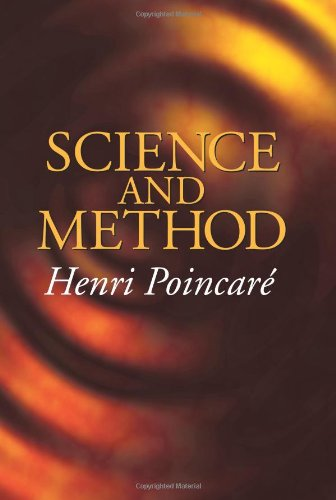# Science and Method by Jules Henri Poincare (Author)_____________________________

'Science and Method'
​By Jules Henri Poincare (Author)

Purchase Book:

Amazon.com
Amazon.co.uk

Description:

This classic by the famous mathematician defines the basic methodology and psychology of scientific discovery, particularly regarding mathematics and mathematical physics. Drawing on examples from many fields, it explains how scientists analyze and choose their working facts, and it explores the nature of experimentation, theory, and the mind. 1914 edition.

________________________________________________

Jules Henri Poincaré (French:29 April 1854 – 17 July 1912) was a French mathematician, theoretical physicist, engineer, and a philosopher of science. He is often described as a polymath, and in mathematics as The Last Universalist by Eric Temple Bell, since he excelled in all fields of the discipline as it existed during his lifetime. As a mathematician and physicist, he made many original fundamental contributions to pure and applied mathematics, mathematical physics, and celestial mechanics. He was responsible for formulating the Poincaré conjecture, which was one of the most famous unsolved problems in mathematics until it was solved in 2002–2003. In his research on the three-body problem, Poincaré became the first person to discover a chaotic deterministic system which laid the foundations of modern chaos theory. He is also considered to be one of the founders of the field of topology. Poincaré made clear the importance of paying attention to the invariance of laws of physics under different transformations, and was the first to present the Lorentz transformations in their modern symmetrical form. Poincaré discovered the remaining relativistic velocity transformations and recorded them in a letter to Dutch physicist Hendrik Lorentz (1853–1928) in 1905. Thus he obtained perfect invariance of all of Maxwell's equations, an important step in the formulation of the theory of special relativity. The Poincaré group used in physics and mathematics was named after him.

_____________________________

_____________________________